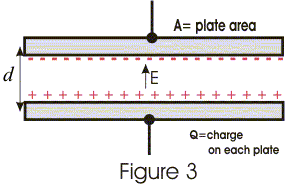# Parallel plate capacitor

## Parallel plate capacitor

• A parallel plate capacitor consists of two large plane parallel conducting plates separated by a small distance shown below in the figure 3.• Suppose two plates of the capacitor has equal and opposite charge Q on them. If A is the area of each plate then surface charge density on each plate is
σ=Q/A
• We have already calculated field between two oppositely charged plates using gauss's law which is
E=σ/ε0=Q/ε0A
and in this result effects near the edges of the plates have been neglected.
• Since electric field between the plates is uniform the potential difference between the plates is
V=Ed=Qd/ε0A
where , d is the separation between the plates.
• Thus, capacitance of parallel plate capacitor in vacuum is
C=Q/V=ε0A/d               (3)
• From equation 3 we see that quantities on which capacitance of parallel plate capacitor depends i.e.,ε0 , A and d are all constants for a capacitor.
• Thus we see that in this case capacitance is independent of charge on the capacitor but depends on area of it's plates and separation distance between the plates.

Question
A parallel plate capacitor has the capacitance of 20 μ F where the distance between the plates is 16 cm.If the distance between the plates is reduced to 4 cm,its capacitance will be
(a) 20 μ F
(b) 5 μ F
(c) 60 μ F
(d) 80 μ F
Solution
$C \alpha \frac {1}{d}$
Therefore
$\frac {C_1}{C_2} = \frac {16}{4}=4$
or
$C_1 = 4 C_2= 80$ μ F